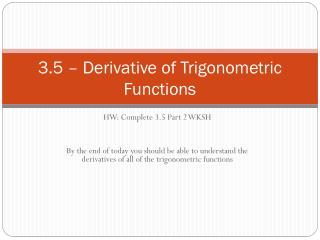DownloadDownload Presentation3.5 – Derivative of Trigonometric Functions

# 3.5 – Derivative of Trigonometric Functions

Télécharger la présentation## 3.5 – Derivative of Trigonometric Functions

- - - - - - - - - - - - - - - - - - - - - - - - - - - E N D - - - - - - - - - - - - - - - - - - - - - - - - - - -
##### Presentation Transcript

1. 3.5 – Derivative of Trigonometric Functions HW: Complete 3.5 Part 2 WKSH By the end of today you should be able to understand the derivatives of all of the trigonometric functions

2. Derivative of sine

3. Derivative of cosine

4. Derivative of tangent

5. Derivative of secant

6. Derivative of cosecant

7. Derivative of cotangent

8. Derivatives of the All the Trigonometric Functions

9. Example 1 • Find the derivatives of • y = x2sinx • v = cosx/(1 – sinx)

10. DEFINITIONJerk Jerk is the derivative of acceleration. If a body’s position at time t is s(t), the body’s jerk at time t is

11. Find y’’ if y = secx